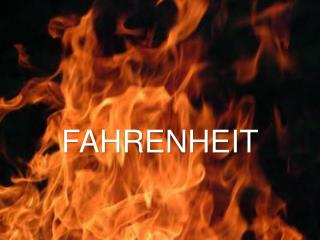DownloadDownload PresentationFAHRENHEIT

# FAHRENHEIT

Download Presentation## FAHRENHEIT

- - - - - - - - - - - - - - - - - - - - - - - - - - - E N D - - - - - - - - - - - - - - - - - - - - - - - - - - -
##### Presentation Transcript

1. FAHRENHEIT

2. Instructions:Take a look at these hot questions about heat. Get the most right and cool down with some donuts! (All answers will be in Fahrenheit degrees…)

3. FAHRENHEIT QUESTIONS

4. #1 How hot is the surface of the sun? A. 10,000 degrees B. 12,000 degrees C. 15,000 degrees

5. #2 A book was published in 1953 with ‘Fahrenheit’ in the title. What was the temperature that accompanied it? A. 32 degrees B. 212 degrees C. 451 degrees

6. #3 At what temperature does water boil? A. 32 degrees B. 212 degrees C. 300 degrees

7. #4 What is Miami’s average temperature for July? A. 84 degrees B. 89 degrees C. 91 degrees

8. #5 At what temperature does lead melt? A. 584 degrees B. 621 degrees C. 632 degrees

9. #6 The Fahrenheit system was named after an 18th century scientist. What was his first name? A. Francis B. Daniel C. Alexander

10. #7 What is Chicago’s average temperature in January? A. 26 degrees B. 29 degrees C. 32 degrees

11. #8 The hottest ever recorded temperature on earth was in Iran. How hot was it? A. 98 degrees B. 122 degrees C. 159 degrees

12. #9 Which of these colors is the “hottest”? A. red B. white C. blue

13. #10 If your temperature is 50 degrees Celsius, how hot is that in Fahrenheit? A. 89 degrees B. 122 degrees C. 190 degrees

15. #1 How hot is the surface of the sun? A. 10,000 degrees B. 12,000 degrees C. 15,000 degrees

16. #1 How hot is the surface of the sun? A. 10,000 degrees

17. #2 A book was published in 1953 with ‘Fahrenheit’ in the title. What was the temperature that accompanied it? A. 32 degrees B. 212 degrees C. 451 degrees

18. #2 A book was published in 1953 with ‘Fahrenheit’ in the title. What was the temperature that accompanied it? C. 451 degrees

19. #3 At what temperature does water boil? A. 32 degrees B. 212 degrees C. 300 degrees

20. #3 At what temperature does water boil? B. 212 degrees

21. #4 What is Miami’s average temperature for July? A. 84 degrees B. 89 degrees C. 91 degrees

22. #4 What is Miami’s average temperature for July? C. 91 degrees

23. #5 At what temperature does lead melt? A. 584 degrees B. 621 degrees C. 632 degrees

24. #5 At what temperature does lead melt? B. 621 degrees

25. #6 The Fahrenheit system was named after an 18th century scientist. What was his first name? A. Francis B. Daniel C. Alexander

26. #6 The Fahrenheit system was named after an 18th century scientist. What was his first name? B. Daniel

27. #7 What is Chicago’s average temperature in January? A. 26 degrees B. 29 degrees C. 32 degrees

28. #7 What is Chicago’s average temperature in January? C. 32 degrees

29. #8 The hottest ever recorded temperature on earth was in Iran. How hot was it? A. 98 degrees B. 122 degrees C. 159 degrees

30. #8 The hottest ever recorded temperature on earth was in Iran. How hot was it? C. 159 degrees

31. #9 Which of these colors is the “hottest”? A. red B. white C. blue

32. #9 Which of these colors is the “hottest”? C. blue

33. #10 If your temperature is 50 degrees Celsius, how hot is that in Fahrenheit? A. 89 degrees B. 122 degrees C. 190 degrees

34. #10 If your temperature is 50 degrees Celsius, how hot is that in Fahrenheit? B. 122 degrees

35. FAHRENHEIT TIE-BREAKERS

36. #TB1 What temperature is called “absolute zero”? A. -189.01 degrees B. -334.89 degrees C. -459.67 degrees

37. FAHRENHEIT TIE-BREAKERS

38. #TB1 What temperature is called “absolute zero”? A. -189.01 degrees B. -334.89 degrees C. -459.67 degrees

39. #TB1 What temperature is called “absolute zero”? C. -459.67 degrees

40. FAHRENHEIT TIE-BREAKERS

41. #TB2 What year did the Fahrenheit system begin? A. 1492 B. 1717 C. 1724

42. FAHRENHEIT TIE-BREAKERS

43. #TB2 What year did the Fahrenheit system begin? A. 1492 B. 1717 C. 1724

44. #TB2 What year did the Fahrenheit system begin? C. 1724

45. FAHRENHEIT TIE-BREAKERS

46. #TB3 Pick the hot number between 1 and 50.

47. FAHRENHEIT TIE-BREAKERS

48. #TB3 Pick the hot number between 1 and 50.

49. #TB3 Pick the hot number between 1 and 50. 33

50. FAHRENHEIT